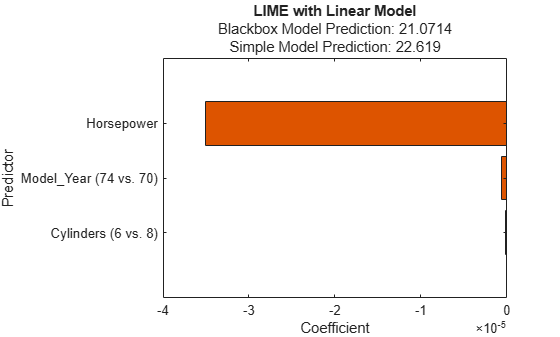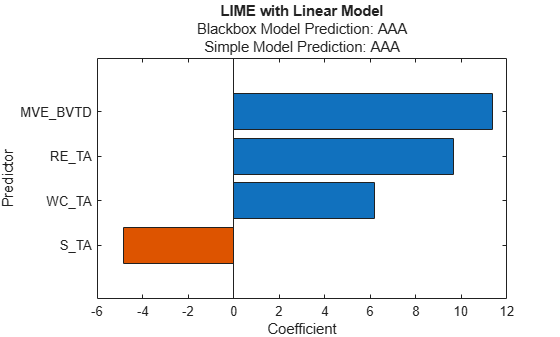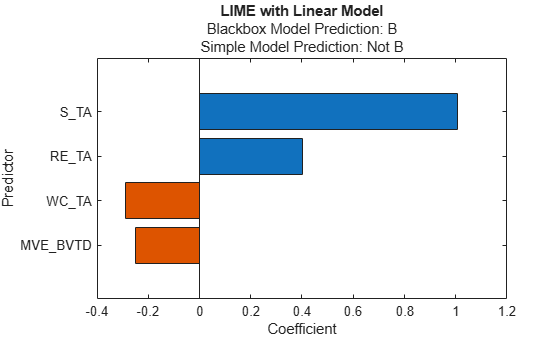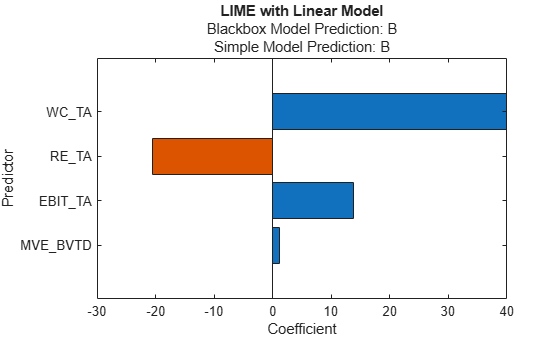# fit

Fit simple model of local interpretable model-agnostic explanations (LIME)

Since R2020b

## Syntax

``newresults = fit(results,queryPoint,numImportantPredictors)``
``newresults = fit(results,queryPoint,numImportantPredictors,Name,Value)``

## Description

example

````newresults = fit(results,queryPoint,numImportantPredictors)` fits a new simple model for the specified query point (`queryPoint`) by using the specified number or predictors (`numImportantPredictors`). The function returns a `lime` object `newresults` that contains the new simple model.`fit` uses the simple model options that you specify when you create the `lime` object `results`. You can change the options using the name-value pair arguments of the `fit` function.```

example

````newresults = fit(results,queryPoint,numImportantPredictors,Name,Value)` specifies additional options using one or more name-value pair arguments. For example, you can specify `'SimpleModelType','tree'` to fit a decision tree model.```

## Examples

collapse all

Train a regression model and create a `lime` object that uses a linear simple model. When you create a `lime` object, if you do not specify a query point and the number of important predictors, then the software generates samples of a synthetic data set but does not fit a simple model. Use the object function `fit` to fit a simple model for a query point. Then display the coefficients of the fitted linear simple model by using the object function `plot`.

Load the `carbig` data set, which contains measurements of cars made in the 1970s and early 1980s.

`load carbig`

Create a table containing the predictor variables `Acceleration`, `Cylinders`, and so on, as well as the response variable `MPG`.

`tbl = table(Acceleration,Cylinders,Displacement,Horsepower,Model_Year,Weight,MPG);`

Removing missing values in a training set can help reduce memory consumption and speed up training for the `fitrkernel` function. Remove missing values in `tbl`.

`tbl = rmmissing(tbl);`

Create a table of predictor variables by removing the response variable from `tbl`.

`tblX = removevars(tbl,'MPG');`

Train a blackbox model of `MPG` by using the `fitrkernel` function.

```rng('default') % For reproducibility mdl = fitrkernel(tblX,tbl.MPG,'CategoricalPredictors',[2 5]);```

Create a `lime` object. Specify a predictor data set because `mdl` does not contain predictor data.

`results = lime(mdl,tblX)`
```results = lime with properties: BlackboxModel: [1x1 RegressionKernel] DataLocality: 'global' CategoricalPredictors: [2 5] Type: 'regression' X: [392x6 table] QueryPoint: [] NumImportantPredictors: [] NumSyntheticData: 5000 SyntheticData: [5000x6 table] Fitted: [5000x1 double] SimpleModel: [] ImportantPredictors: [] BlackboxFitted: [] SimpleModelFitted: [] ```

`results` contains the generated synthetic data set. The `SimpleModel` property is empty (`[]`).

Fit a linear simple model for the first observation in `tblX`. Specify the number of important predictors to find as 3.

`queryPoint = tblX(1,:)`
```queryPoint=1×6 table Acceleration Cylinders Displacement Horsepower Model_Year Weight ____________ _________ ____________ __________ __________ ______ 12 8 307 130 70 3504 ```
`results = fit(results,queryPoint,3);`

Plot the `lime` object `results` by using the object function `plot`. To display an existing underscore in any predictor name, change the `TickLabelInterpreter` value of the axes to `'none'`.

```f = plot(results); f.CurrentAxes.TickLabelInterpreter = 'none';```The plot displays two predictions for the query point, which correspond to the BlackboxFitted property and the SimpleModelFitted property of `results`.

The horizontal bar graph shows the coefficient values of the simple model, sorted by their absolute values. LIME finds `Horsepower`, `Model_Year`, and `Cylinders` as important predictors for the query point.

`Model_Year` and `Cylinders` are categorical predictors that have multiple categories. For a linear simple model, the software creates one less dummy variable than the number of categories for each categorical predictor. The bar graph displays only the most important dummy variable. You can check the coefficients of the other dummy variables using the `SimpleModel` property of `results`. Display the sorted coefficient values, including all categorical dummy variables.

```[~,I] = sort(abs(results.SimpleModel.Beta),'descend'); table(results.SimpleModel.ExpandedPredictorNames(I)',results.SimpleModel.Beta(I), ... 'VariableNames',{'Expanded Predictor Name','Coefficient'})```
```ans=17×2 table Expanded Predictor Name Coefficient __________________________ ___________ {'Cylinders (5 vs. 8)' } 0.18008 {'Model_Year (74 vs. 70)'} -0.082499 {'Model_Year (80 vs. 70)'} -0.052277 {'Model_Year (81 vs. 70)'} 0.035987 {'Model_Year (82 vs. 70)'} -0.026442 {'Model_Year (71 vs. 70)'} 0.014736 {'Model_Year (76 vs. 70)'} 0.014723 {'Model_Year (75 vs. 70)'} 0.013979 {'Model_Year (77 vs. 70)'} 0.012762 {'Model_Year (78 vs. 70)'} 0.0089647 {'Cylinders (6 vs. 8)' } -0.006972 {'Model_Year (79 vs. 70)'} -0.0058682 {'Model_Year (72 vs. 70)'} 0.005654 {'Cylinders (3 vs. 8)' } -0.0023194 {'Horsepower' } -0.00021074 {'Cylinders (4 vs. 8)' } 0.00014773 ⋮ ```

Train a classification model and create a `lime` object that uses a decision tree simple model. Fit multiple models for multiple query points.

Load the `CreditRating_Historical` data set. The data set contains customer IDs and their financial ratios, industry labels, and credit ratings.

`tbl = readtable('CreditRating_Historical.dat');`

Create a table of predictor variables by removing the columns of customer IDs and ratings from `tbl`.

`tblX = removevars(tbl,["ID","Rating"]);`

Train a blackbox model of credit ratings by using the `fitcecoc` function.

`blackbox = fitcecoc(tblX,tbl.Rating,'CategoricalPredictors','Industry')`
```blackbox = ClassificationECOC PredictorNames: {'WC_TA' 'RE_TA' 'EBIT_TA' 'MVE_BVTD' 'S_TA' 'Industry'} ResponseName: 'Y' CategoricalPredictors: 6 ClassNames: {'A' 'AA' 'AAA' 'B' 'BB' 'BBB' 'CCC'} ScoreTransform: 'none' BinaryLearners: {21x1 cell} CodingName: 'onevsone' Properties, Methods ```

Create a `lime` object with the `blackbox` model.

```rng('default') % For reproducibility results = lime(blackbox);```

Find two query points whose true rating values are `AAA` and `B`, respectively.

```queryPoint(1,:) = tblX(find(strcmp(tbl.Rating,'AAA'),1),:); queryPoint(2,:) = tblX(find(strcmp(tbl.Rating,'B'),1),:)```
```queryPoint=2×6 table WC_TA RE_TA EBIT_TA MVE_BVTD S_TA Industry _____ _____ _______ ________ _____ ________ 0.121 0.413 0.057 3.647 0.466 12 0.019 0.009 0.042 0.257 0.119 1 ```

Fit a linear simple model for the first query point. Set the number of important predictors to 4.

`newresults1 = fit(results,queryPoint(1,:),4);`

Plot the LIME results `newresults1` for the first query point. To display an existing underscore in any predictor name, change the `TickLabelInterpreter` value of the axes to `'none'`.

```f1 = plot(newresults1); f1.CurrentAxes.TickLabelInterpreter = 'none';```Fit a linear decision tree model for the first query point.

```newresults2 = fit(results,queryPoint(1,:),6,'SimpleModelType','tree'); f2 = plot(newresults2); f2.CurrentAxes.TickLabelInterpreter = 'none';```The simple models in `newresults1` and `newresults2` both find `MVE_BVTD` and `RE_TA` as important predictors.

Fit a linear simple model for the second query point, and plot the LIME results for the second query point.

```newresults3 = fit(results,queryPoint(2,:),4); f3 = plot(newresults3); f3.CurrentAxes.TickLabelInterpreter = 'none';```The prediction from the `blackbox` model is `B`, but the prediction from the simple model is not `B`. When the two predictions are not the same, you can specify a smaller `'KernelWidth'` value. The software fits a simple model using weights that are more focused on the samples near the query point. If a query point is an outlier or is located near a decision boundary, then the two prediction values can be different, even if you specify a small `'KernelWidth'` value. In such a case, you can change other name-value pair arguments. For example, you can generate a local synthetic data set (specify `'DataLocality'` of `lime` as `'local'`) for the query point and increase the number of samples (`'NumSyntheticData'` of `lime` or `fit`) in the synthetic data set. You can also use a different distance metric (`'Distance'` of `lime` or `fit`).

Fit a linear simple model with a small `'KernelWidth'` value.

```newresults4 = fit(results,queryPoint(2,:),4,'KernelWidth',0.01); f4 = plot(newresults4); f4.CurrentAxes.TickLabelInterpreter = 'none';```The credit ratings for the first and second query points are `AAA` and `B`, respectively. The simple models in `newresults1` and `newresults4` both find `MVE_BVTD`, `RE_TA`, and `WC_TA` as important predictors. However, their coefficient values are different. The plots show that these predictors act differently depending on the credit ratings.

## Input Arguments

collapse all

LIME results, specified as a `lime` object.

Query point around which the `fit` function fits the simple model, specified as a row vector of numeric values or a single-row table. The `queryPoint` value must have the same data type and the same number of columns as the predictor data (`results.X` or `results.SyntheticData`) in the `lime` object `results`.

`queryPoint` must not contain missing values.

Data Types: `single` | `double` | `table`

Number of important predictors to use in the simple model, specified as a positive integer scalar value.

• If `'SimpleModelType'` is `'linear'`, then the software selects the specified number of important predictors and fits a linear model of the selected predictors.

• If `'SimpleModelType'` is `'tree'`, then the software specifies the maximum number of decision splits (or branch nodes) as the number of important predictors so that the fitted decision tree uses at most the specified number of predictors.

The default value of the `numImportantPredictors` argument is the `NumImportantPredictors` property value of the `lime` object `results`. If you do not specify the property value when creating `results`, then the property value is empty (`[]`) and you must specify this argument.

Data Types: `single` | `double`

### Name-Value Arguments

Specify optional pairs of arguments as `Name1=Value1,...,NameN=ValueN`, where `Name` is the argument name and `Value` is the corresponding value. Name-value arguments must appear after other arguments, but the order of the pairs does not matter.

Before R2021a, use commas to separate each name and value, and enclose `Name` in quotes.

Example: `'NumSyntheticData',2000,'SimpleModelType','tree'` sets the number of samples to generate for the synthetic data set to 2000 and specifies the simple model type as a decision tree.

Covariance matrix for the Mahalanobis distance metric, specified as the comma-separated pair consisting of `'Cov'` and a K-by-K positive definite matrix, where K is the number of predictors.

This argument is valid only if `'Distance'` is `'mahalanobis'`.

The default value is the `'Cov'` value that you specify when creating the `lime` object `results`. The default `'Cov'` value of `lime` is `cov(PD,'omitrows')`, where `PD` is the predictor data or synthetic predictor data. If you do not specify the `'Cov'` value, then the software uses different covariance matrices when computing the distances for both the predictor data and the synthetic predictor data.

Example: `'Cov',eye(3)`

Data Types: `single` | `double`

Distance metric, specified as the comma-separated pair consisting of `'Distance'` and a character vector, string scalar, or function handle.

• If the predictor data includes only continuous variables, then `fit` supports these distance metrics.

ValueDescription
`'euclidean'`

Euclidean distance.

`'seuclidean'`

Standardized Euclidean distance. Each coordinate difference between observations is scaled by dividing by the corresponding element of the standard deviation, ```S = std(PD,'omitnan')```, where `PD` is the predictor data or synthetic predictor data. To specify different scaling, use the `'Scale'` name-value argument.

`'mahalanobis'`

Mahalanobis distance using the sample covariance of `PD`, ```C = cov(PD,'omitrows')```. To change the value of the covariance matrix, use the `'Cov'` name-value argument.

`'cityblock'`

City block distance.

`'minkowski'`

Minkowski distance. The default exponent is 2. To specify a different exponent, use the `'P'` name-value argument.

`'chebychev'`

Chebychev distance (maximum coordinate difference).

`'cosine'`

One minus the cosine of the included angle between points (treated as vectors).

`'correlation'`

One minus the sample correlation between points (treated as sequences of values).

`'spearman'`

One minus the sample Spearman's rank correlation between observations (treated as sequences of values).

`@distfun`

Custom distance function handle. A distance function has the form

```function D2 = distfun(ZI,ZJ) % calculation of distance ...```
where

• `ZI` is a `1`-by-`t` vector containing a single observation.

• `ZJ` is an `s`-by-`t` matrix containing multiple observations. `distfun` must accept a matrix `ZJ` with an arbitrary number of observations.

• `D2` is an `s`-by-`1` vector of distances, and `D2(k)` is the distance between observations `ZI` and `ZJ(k,:)`.

If your data is not sparse, you can generally compute distance more quickly by using a built-in distance metric instead of a function handle.

• If the predictor data includes both continuous and categorical variables, then `fit` supports these distance metrics.

ValueDescription
`'goodall3'`

Modified Goodall distance

`'ofd'`

Occurrence frequency distance

For definitions, see Distance Metrics.

The default value is the `'Distance'` value that you specify when creating the `lime` object `results`. The default `'Distance'` value of `lime` is `'euclidean'` if the predictor data includes only continuous variables, or `'goodall3'` if the predictor data includes both continuous and categorical variables.

Example: `'Distance','ofd'`

Data Types: `char` | `string` | `function_handle`

Kernel width of the squared exponential (or Gaussian) kernel function, specified as the comma-separated pair consisting of `'KernelWidth'` and a numeric scalar value.

The `fit` function computes distances between the query point and the samples in the synthetic predictor data set, and then converts the distances to weights by using the squared exponential kernel function. If you lower the `'KernelWidth'` value, then `fit` uses weights that are more focused on the samples near the query point. For details, see LIME.

The default value is the `'KernelWidth'` value that you specify when creating the `lime` object `results`. The default `'KernelWidth'` value of `lime` is 0.75.

Example: `'KernelWidth',0.5`

Data Types: `single` | `double`

Number of neighbors of the query point, specified as the comma-separated pair consisting of `'NumNeighbors'` and a positive integer scalar value. This argument is valid only when the `DataLocality` property of `results` is `'local'`.

The `fit` function estimates the distribution parameters of the predictor data using the specified number of nearest neighbors of the query point. Then the function generates synthetic predictor data using the estimated distribution.

If you specify a value larger than the number of observations in the predictor data set (`results.X`) in the `lime` object `results`, then `fit` uses all observations.

The default value is the `'NumNeighbors'` value that you specify when creating the `lime` object `results`. The default `'NumNeighbors'` value of `lime` is 1500.

Example: `'NumNeighbors',2000`

Data Types: `single` | `double`

Number of samples to generate for the synthetic data set, specified as the comma-separated pair consisting of `'NumSyntheticData'` and a positive integer scalar value.

The default value is the `NumSyntheticData` property value of the `lime` object `results`. If you provide a custom synthetic data set when creating `results`, then the property value is the number of samples in the data set. Otherwise, the `'NumSyntheticData'` value that you specify when creating `results` sets the property. The default `'NumSyntheticData'` value of `lime` is 5000.

Example: `'NumSyntheticData',2500`

Data Types: `single` | `double`

Exponent for the Minkowski distance metric, specified as the comma-separated pair consisting of `'P'` and a positive scalar.

This argument is valid only if `'Distance'` is `'minkowski'`.

The default value is the `'P'` value that you specify when creating the `lime` object `results`. The default `'P'` value of `lime` is 2.

Example: `'P',3`

Data Types: `single` | `double`

Scale parameter value for the standardized Euclidean distance metric, specified as the comma-separated pair consisting of `'Scale'` and a nonnegative numeric vector of length K, where K is the number of predictors.

This argument is valid only if `'Distance'` is `'seuclidean'`.

The default value is the `'Scale'` value that you specify when creating the `lime` object `results`. The default `'Scale'` value of `lime` is `std(PD,'omitnan')`, where `PD` is the predictor data or synthetic predictor data. If you do not specify the `'Scale'` value, then the software uses different scale parameters when computing the distances for both the predictor data and the synthetic predictor data.

Example: ```'Scale',quantile(X,0.75) - quantile(X,0.25)```

Data Types: `single` | `double`

Type of the simple model, specified as the comma-separated pair consisting of `'SimpleModelType'` and `'linear'` or `'tree'`.

The default value is the `'SimpleModelType'` value that you specify when creating the `lime` object `results`. The default `'SimpleModelType'` value of `lime` is `'linear'`.

Example: `'SimpleModelType','tree'`

Data Types: `char` | `string`

## Output Arguments

collapse all

LIME results, returned as a `lime` object. `newresults` contains the new simple model.

To overwrite the input argument `results`, assign the output of `fit` to `results`:

`results = fit(results,queryPoint,numImportantPredictors);`

collapse all

### Distance Metrics

A distance metric is a function that defines a distance between two observations. `fit` supports various distance metrics for continuous variables and a mix of continuous and categorical variables.

• Distance metrics for continuous variables

Given an mx-by-n data matrix X, which is treated as mx (1-by-n) row vectors x1, x2, ..., xmx, and an my-by-n data matrix Y, which is treated as my (1-by-n) row vectors y1, y2, ...,ymy, the various distances between the vector xs and yt are defined as follows:

• Euclidean distance

`${d}_{st}^{2}=\left({x}_{s}-{y}_{t}\right)\left({x}_{s}-{y}_{t}{\right)}^{\prime }.$`

The Euclidean distance is a special case of the Minkowski distance, where p = 2.

• Standardized Euclidean distance

`${d}_{st}^{2}=\left({x}_{s}-{y}_{t}\right){V}^{-1}\left({x}_{s}-{y}_{t}{\right)}^{\prime },$`

where V is the n-by-n diagonal matrix whose jth diagonal element is (S(j))2, where S is a vector of scaling factors for each dimension.

• Mahalanobis distance

`${d}_{st}^{2}=\left({x}_{s}-{y}_{t}\right){C}^{-1}\left({x}_{s}-{y}_{t}{\right)}^{\prime },$`

where C is the covariance matrix.

• City block distance

`${d}_{st}=\sum _{j=1}^{n}|{x}_{sj}-{y}_{tj}|.$`

The city block distance is a special case of the Minkowski distance, where p = 1.

• Minkowski distance

`${d}_{st}=\sqrt[p]{\sum _{j=1}^{n}{|{x}_{sj}-{y}_{tj}|}^{p}}.$`

For the special case of p = 1, the Minkowski distance gives the city block distance. For the special case of p = 2, the Minkowski distance gives the Euclidean distance. For the special case of p = ∞, the Minkowski distance gives the Chebychev distance.

• Chebychev distance

`${d}_{st}={\mathrm{max}}_{j}\left\{|{x}_{sj}-{y}_{tj}|\right\}.$`

The Chebychev distance is a special case of the Minkowski distance, where p = ∞.

• Cosine distance

`${d}_{st}=\left(1-\frac{{x}_{s}{{y}^{\prime }}_{t}}{\sqrt{\left({x}_{s}{{x}^{\prime }}_{s}\right)\left({y}_{t}{{y}^{\prime }}_{t}\right)}}\right).$`
• Correlation distance

`${d}_{st}=1-\frac{\left({x}_{s}-{\overline{x}}_{s}\right){\left({y}_{t}-{\overline{y}}_{t}\right)}^{\prime }}{\sqrt{\left({x}_{s}-{\overline{x}}_{s}\right){\left({x}_{s}-{\overline{x}}_{s}\right)}^{\prime }}\sqrt{\left({y}_{t}-{\overline{y}}_{t}\right){\left({y}_{t}-{\overline{y}}_{t}\right)}^{\prime }}},$`

where

`${\overline{x}}_{s}=\frac{1}{n}\sum _{j}{x}_{sj}$`

and

`${\overline{y}}_{t}=\frac{1}{n}\sum _{j}{y}_{tj}.$`
• Spearman distance

`${d}_{st}=1-\frac{\left({r}_{s}-{\overline{r}}_{s}\right){\left({r}_{t}-{\overline{r}}_{t}\right)}^{\prime }}{\sqrt{\left({r}_{s}-{\overline{r}}_{s}\right){\left({r}_{s}-{\overline{r}}_{s}\right)}^{\prime }}\sqrt{\left({r}_{t}-{\overline{r}}_{t}\right){\left({r}_{t}-{\overline{r}}_{t}\right)}^{\prime }}},$`

where

• rsj is the rank of xsj taken over x1j, x2j, ...xmx,j, as computed by `tiedrank`.

• rtj is the rank of ytj taken over y1j, y2j, ...ymy,j, as computed by `tiedrank`.

• rs and rt are the coordinate-wise rank vectors of xs and yt, that is, rs = (rs1, rs2, ... rsn) and rt = (rt1, rt2, ... rtn).

• ${\overline{r}}_{s}=\frac{1}{n}\sum _{j}{r}_{sj}=\frac{\left(n+1\right)}{2}$.

• ${\overline{r}}_{t}=\frac{1}{n}\sum _{j}{r}_{tj}=\frac{\left(n+1\right)}{2}$.

• Distance metrics for a mix of continuous and categorical variables

• Modified Goodall distance

This distance is a variant of the Goodall distance, which assigns a small distance if the matching values are infrequent regardless of the frequencies of the other values. For mismatches, the distance contribution of the predictor is 1/(number of variables).

• Occurrence frequency distance

For a match, the occurrence frequency distance assigns zero distance. For a mismatch, the occurrence frequency distance assigns a higher distance on a less frequent value and a lower distance on a more frequent value.

## Algorithms

collapse all

### LIME

To explain a prediction of a machine learning model using LIME , the software generates a synthetic data set and fits a simple interpretable model to the synthetic data set by using `lime` and `fit`, as described in steps 1–5.

• If you specify the `queryPoint` and `numImportantPredictors` values of `lime`, then the `lime` function performs all steps.

• If you do not specify `queryPoint` and `numImportantPredictors` and specify `'DataLocality'` as `'global'` (default), then the `lime` function generates a synthetic data set (steps 1–2), and the `fit` function fits a simple model (steps 3–5).

• If you do not specify `queryPoint` and `numImportantPredictors` and specify `'DataLocality'` as `'local'`, then the `fit` function performs all steps.

The `lime` and `fit` functions perform these steps:

1. Generate a synthetic predictor data set Xs using a multivariate normal distribution for continuous variables and a multinomial distribution for each categorical variable. You can specify the number of samples to generate by using the `'NumSyntheticData'` name-value argument.

• If `'DataLocality'` is `'global'` (default), then the software estimates the distribution parameters from the whole predictor data set (`X` or predictor data in `blackbox`).

• If `'DataLocality'` is `'local'`, then the software estimates the distribution parameters using the k-nearest neighbors of the query point, where k is the `'NumNeighbors'` value. You can specify a distance metric to find the nearest neighbors by using the `'Distance'` name-value argument.

The software ignores missing values in the predictor data set when estimating the distribution parameters.

Alternatively, you can provide a pregenerated, custom synthetic predictor data set by using the `customSyntheticData` input argument of `lime`.

2. Compute the predictions Ys for the synthetic data set Xs. The predictions are predicted responses for regression or classified labels for classification. The software uses the `predict` function of the `blackbox` model to compute the predictions. If you specify `blackbox` as a function handle, then the software computes the predictions by using the function handle.

3. Compute the distances d between the query point and the samples in the synthetic predictor data set using the distance metric specified by `'Distance'`.

4. Compute the weight values wq of the samples in the synthetic predictor data set with respect to the query point q using the squared exponential (or Gaussian) kernel function

`${w}_{q}\left({x}_{s}\right)=\mathrm{exp}\left(-\frac{1}{2}{\left(\frac{d\left({x}_{s},q\right)}{\sqrt{p}\text{ }\sigma }\right)}^{2}\right).$`

• xs is a sample in the synthetic predictor data set Xs.

• d(xs,q) is the distance between the sample xs and the query point q.

• p is the number of predictors in Xs.

• σ is the kernel width, which you can specify by using the `'KernelWidth'` name-value argument. The default `'KernelWidth'` value is 0.75.

The weight value at the query point is 1, and then it converges to zero as the distance value increases. The `'KernelWidth'` value controls how fast the weight value converges to zero. The lower the `'KernelWidth'` value, the faster the weight value converges to zero. Therefore, the algorithm gives more weight to samples near the query point. Because this algorithm uses such weight values, the selected important predictors and fitted simple model effectively explain the predictions for the synthetic data locally, around the query point.

5. Fit a simple model.

• If `'SimpleModelType'` is `'linear'` (default), then the software selects important predictors and fits a linear model of the selected important predictors.

• Select n important predictors (${\stackrel{˜}{X}}_{s}$) by using the group orthogonal matching pursuit (OMP) algorithm , where n is the `numImportantPredictors` value. This algorithm uses the synthetic predictor data set (Xs), predictions (Ys), and weight values (wq).

• Fit a linear model of the selected important predictors (${\stackrel{˜}{X}}_{s}$) to the predictions (Ys) using the weight values (wq). The software uses `fitrlinear` for regression or `fitclinear` for classification. For a multiclass model, the software uses the one-versus-all scheme to construct a binary classification problem. The positive class is the predicted class for the query point from the `blackbox` model, and the negative class refers to the other classes.

• If `'SimpleModelType'` is `'tree'`, then the software fits a decision tree model by using `fitrtree` for regression or `fitctree` for classification. The software specifies the maximum number of decision splits (or branch nodes) as the number of important predictors so that the fitted decision tree uses at most the specified number of predictors.

 Ribeiro, Marco Tulio, S. Singh, and C. Guestrin. "'Why Should I Trust You?': Explaining the Predictions of Any Classifier." In Proceedings of the 22nd ACM SIGKDD International Conference on Knowledge Discovery and Data Mining, 1135–44. San Francisco, California: ACM, 2016.

 Świrszcz, Grzegorz, Naoki Abe, and Aurélie C. Lozano. "Grouped Orthogonal Matching Pursuit for Variable Selection and Prediction." Advances in Neural Information Processing Systems (2009): 1150–58.

 Lozano, Aurélie C., Grzegorz Świrszcz, and Naoki Abe. "Group Orthogonal Matching Pursuit for Logistic Regression." Proceedings of the Fourteenth International Conference on Artificial Intelligence and Statistics (2011): 452–60.